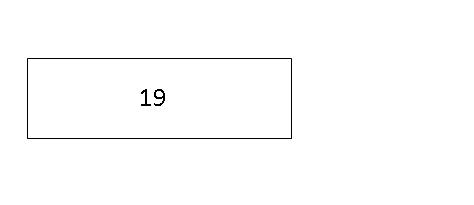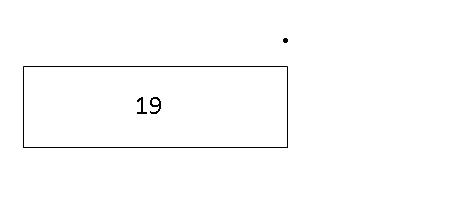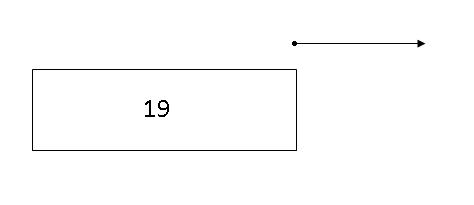The Place Holder Concept

The Place Holder Concept is derived from the Part-Whole Concept . Quite frequently, questions involving the Place-Holder Concept can be easily solved if the place-holder appears on the right side of an addition or subtraction statement.

For example, 19 - 7 = ___

However, if the place-holder were to appear on the left side of the equation, it would present a great challenge to some kids. When children first start learning the four operations of Math, they were taught that the "+" sign means "plus", "add" or "the sum of". Similarly, the "-" sign means "minus", "subtract" or "the difference between".

So it should not surprise you when your kid think that he/she needs to add up the 2 numbers in the following question:

___ + 7 = 19

Likewise, they would think that they should subtract the smaller number from the greater number in the following question:

___ - 7 = 21

If your kids are older or have mastered the concept of Algebra, they would most probably not have any problems with it. However, for younger kids, we can introduce a more concrete approach --- the Model Method.

To illustrate this concept, consider the following questions.

(A) 19 + 7 = ___

Step 1: Always start from the first number on the left side of the equation. Draw a box to represent 19.Step 2: Since the sign after 19 is "+", we are going to add a number to 19, thereby making it a number greater than 19. So, we put a dot at the top-right corner of the first box. This marks the starting point of the arrow we are about to draw next.Step 3: Draw an arrow from the dot pointing to the right. (The arrow points to the right because we are adding another number to the first number to make it greater.)Step 4: Since we are adding 7, we are actually making the number 19 (starting number) greater by 7. Hence, draw a second box directly below the arrow to show that the number 19 has been lengthened by 7.Step 5: Draw a last box to match the length of the first two boxes and write the unknown number (?) in this box.From the model,

19 + 7 = ?

19 + 7 = 26

Thus, the answer is 26.

(B) 19 + ___ = 26

Step 1: Always start from the first number on the left side of the equation. Draw a box to represent 19.Step 2: Since the sign after 19 is "+", we are going to add a number to 19, thereby making it a number greater than 19. So, we put a dot at the top-right corner of the first box. This marks the starting point of the arrow we are about to draw next.Step 3: Draw an arrow from the dot pointing to the right. (The arrow points to the right because we are adding another number to the first number to make it greater.)Step 4: Since we are adding an unknown number, we are actually making the number 19 (starting number) greater by this unknown number. Hence, draw a second box directly below the arrow to show that the number 19 has been lengthened by the unknown number "?".Step 5: Draw a last box to match the length of the first two boxes and write the number 26 in this box.From the model,

26 - 19 = ?

26 - 19 = 7

Thus, the answer is 7.

(C) ___ + 7 = 26

Step 1: Always start from the first number on the left side of the equation. Draw a box to represent the unknown number "?".Step 2: Since the sign after the unknown number "?" is "+", we are going to add a number to "?", thereby making it a number greater than "?". So, we put a dot at the top-right corner of the first box. This marks the starting point of the arrow we are about to draw next.Step 3: Draw an arrow from the dot pointing to the right. (The arrow points to the right because we are adding another number to the first number to make it greater.)Step 4: Since we are adding 7, we are actually making the unknown number "?" (starting number) greater by 7. Hence, draw a second box directly below the arrow to show that the unknown number "?" has been lengthened by 7.Step 5: Draw a last box to match the length of the first two boxes and write the number 26 in this box.From the model,

26 - 7 = ?

26 - 7 = 19

Thus, the answer is 19.

(D) 19 - 7 = ___

Step 1: Always start from the first number on the left side of the equation. Draw a box to represent 19.Step 2: Since the sign after 19 is "-", we are going to subtract a number from 19, thereby making it a number smaller than 19. So, we put a dot at the top-right corner of the first box. This marks the starting point of the arrow we are about to draw next.Step 3: Draw an arrow from the dot pointing to the left. (The arrow points to the left because we are subtracting another number from the first number to make it smaller.)Step 4: Since we subtracting 7, we are actually making the number 19 (starting number) smaller by 7. Hence, draw a second box directly below the arrow to show that the number 19 has been shortened by 7.Step 5: Draw a last box to represent the difference between the first two boxes and write the unknown number (?) in this box.From the model,

19 - 7 = ?

19 - 7 = 12

Thus, the answer is 12.

(E) 19 - ___ = 12

Step 1: Always start from the first number on the left side of the equation. Draw a box to represent 19.Step 2: Since the sign after 19 is "-", we are going to subtract a number from 19, thereby making it a number smaller than 19. So, we put a dot at the top-right corner of the first box. This marks the starting point of the arrow we are about to draw next.Step 3: Draw an arrow from the dot pointing to the left. (The arrow points to the left because we are subtracting another number from the first number to make it smaller.)Step 4: Since we subtracting an unknown number "?", we are actually making the number 19 (starting number) smaller by "?". Hence, draw a second box directly below the arrow to show that the number 19 has been shortened by "?".Step 5: Draw a last box to represent the difference between the first two boxes and write 12 in this box.From the model,

19 - 12 = ?

19 - 12 = 7

Thus, the answer is 7.

(F) ___ - 7 = 12

Step 1: Always start from the first number on the left side of the equation. Draw a box to represent the unknown number "?".

Step 2: Since the sign after the unknown number "?" is "-", we are going to subtract a number from the unknown number "?", thereby making it a number smaller than "?". So, we put a dot at the top-right corner of the first box. This marks the starting point of the arrow we are about to draw next.

Step 3: Draw an arrow from the dot pointing to the left. (The arrow points to the left because we are subtracting another number from the first number to make it smaller.)

Step 4: Since we subtracting 7, we are actually making the unknown number "?" (starting number) smaller by 7. Hence, draw a second box directly below the arrow to show that the unknown number "?" has been shortened by 7.

Step 5: Draw a last box to represent the difference between the first two boxes and write 12 in this box.

From the model,

12 + 7 = ?

12 + 7 = 19

Thus, the answer is 19.

Go To Top - Place Holder Concept

If you want us to send you our future Modelmatics eZine that would inform you on the latest article in Teach Kids Math By Model Method, do an easy sign-up below. Subscription is FREE!

 Enter your E-mail Address Here Enter your Name Here Then Don't worry — your e-mail address is totally secure. I promise to use it only to send you ModelMatics.